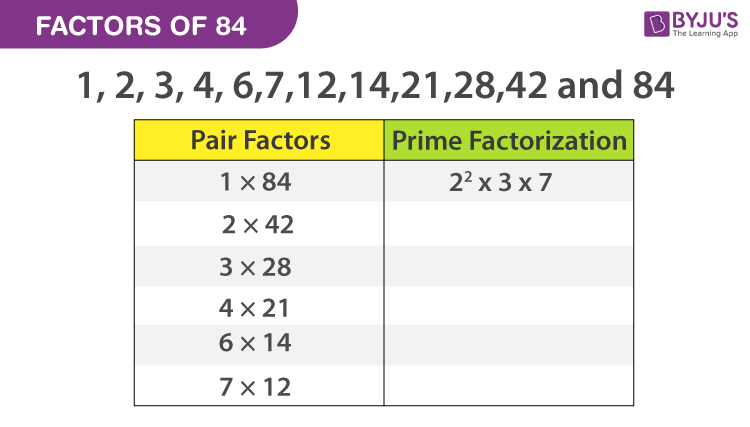Checkout JEE MAINS 2022 Question Paper Analysis : Checkout JEE MAINS 2022 Question Paper Analysis :

# Factors Of 84

Factors of 84 are the numbers that divide the original number evenly. For example, 2 is the factor of 84, since 84 divided by 2 is equal to 42. Pair factors are the numbers which when multiplied in pairs give the original number. For example, 2 and 42 are pair factors. Let us learn to find these factors and pair factors along with prime factors.## Pair Factors of 84

We can find the factor pairs, by multiplying two numbers in a pair to get the original number as 84, such as;

1 × 84 = 84

2 × 42 = 84

3 × 28 = 84

4 × 21 = 84

6 × 14 = 84

7 × 12 = 84

Therefore, the factor pairs are (1, 84), (2, 42), (3, 28), (4, 21), (6, 14) and (7, 12). Thus, with this we can evaluate the unique factors of number 84 as given below;

• Factors of 84 : 1, 2, 3, 4, 6, 7, 12, 14, 21, 28, 42 and 84

We can also write the negative pair factors of 84 since after multiplying the two negative factors, we will get the positive value.

(-1) × (-84) = 84

(-2) × (-42) = 84

(-3) × (-28) = 84

(-4) × (-21) = 84

(-6) × (-14) = 84

(-7) × (-12) = 84

Therefore, the negative pair factors are (-1, -84), (-2, -42), (-3, -28), (-4, -21), (-6, -14) and (-7, -12).

## How to Calculate the Factors of 84?

To find the factors, we need to divide the original number with all the natural numbers till we get the value of the quotient equal to 1.

• 84 ÷ 1 = 84
• 84 ÷ 2 = 42
• 84 ÷ 3 = 28
• 84 ÷ 4 = 21
• 84 ÷ 6 = 14
• 84 ÷ 7 = 12
• 84 ÷ 12 = 7
• 84 ÷ 14 = 6
• 84 ÷ 21 = 4
• 84 ÷ 28 = 3
• 84 ÷ 42 = 2
• 84 ÷ 84 = 1

Therefore, the required factors are: 1, 2, 3, 4, 6, 7, 12, 14, 21, 28, 42 and 84.

### Prime Factorisation of 84

84 is a composite number, so the prime factors of 84 can be found using the below steps.

• The first step is to divide the number 84 with the smallest prime factor, i.e. 2.

84 ÷ 2 = 42

Again, divide 42 by 2.

42 ÷ 2 = 21

Now, if we divide 21 by 2 we get a fraction number, which cannot be a factor.

• Now, proceed to the next prime numbers, i.e. 3, 5, 7 and so on.

21 ÷ 3 = 7

7 ÷ 3 = 2.33, not a factor

Move to next prime number, 5.

• Dividing 7 by 5 again gives a fraction value.

7 ÷ 5 = 1.4, not a factor

Move to next prime number 7.

• Dividing 7 by 7 we get,

7 ÷ 7 = 1

• We have received 1 at the end and further, we cannot proceed with the division. So, the prime factorisation of 84 is 2 × 2 × 3 × 7 or 22 × 3 × 7, where 2, 3 and 7 are the prime numbers.

## Video Lesson on Prime Factors## Solved Examples

Q.1: If Rhea has 84 apples and he has to distribute those to 7 members of the house, including her, then how many apples each of the members get?

Solution: Number of apples, Rhea has = 84

Members in the house including Rhea = 7

Therefore, each member will get = 84/7 = 12 apples.

Q.2: What are the factors of 84 and 114?

Factors of 84 = 1, 2, 3, 4, 6, 7, 12, 14, 21, 28, 42, 84

Factors of 114 = 1, 2, 3, 6, 19, 38, 57, 114

Common factors of 84 and 114 = 1,2,3 and 6.

## Frequently Asked Questions – FAQs

### What are the factors of 84?

The factors of number 84 are 1, 2, 3, 4, 6, 7, 12, 14, 21, 28, 42 and 84.

### Factors and Multiples of 84 are the same. True or False?

False. Factors and multiples of 84 are different and not the same.

### What are the multiples of 84 in Maths?

The multiples of 84 are 84 168 252 336 420 504 588 672 756 and 840.

### What are the common factors of 98 and 84?

Factors of 84 = 1, 2, 3, 4, 6, 7, 12, 14, 21, 28, 42, 84
Factors of 98 = 1, 2, 7, 14, 49, 98
Common factors of 98 and 84 = 1, 2, 7 and 14.

### What is the greatest prime factor of 84?

The prime factorisation of 84 is 2 × 2 × 3 × 7. Therefore, the greatest prime factor is 7.

 Links Related to Factors Factors of 64 Factor of 36 Factors of 48 Factors of 18 Factors of 24 Factors of 25 Factors of 42 Factors of 60 Factors of 35 Factors of 81 Factors of 75 Factors of 56
Test your knowledge on Factors of 84